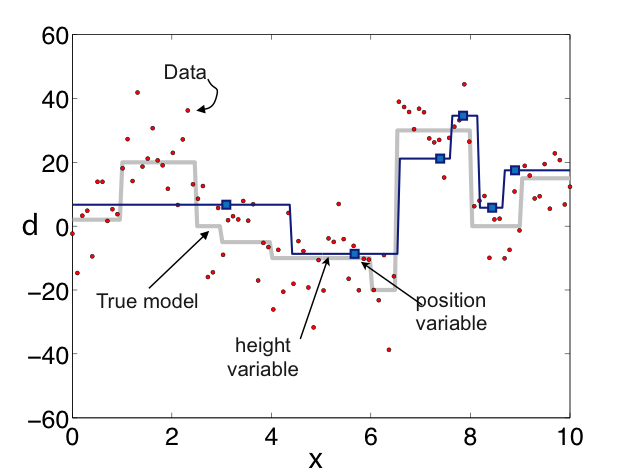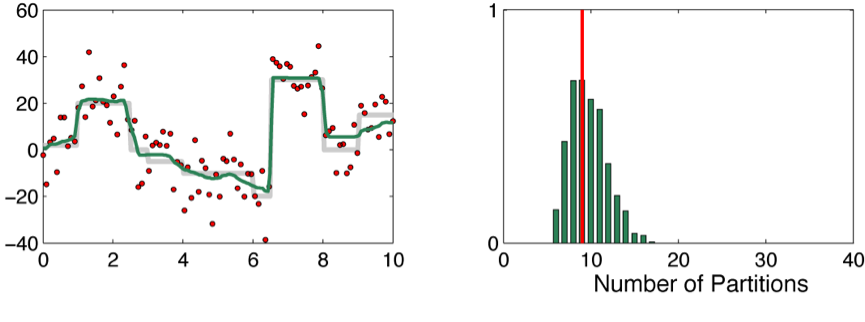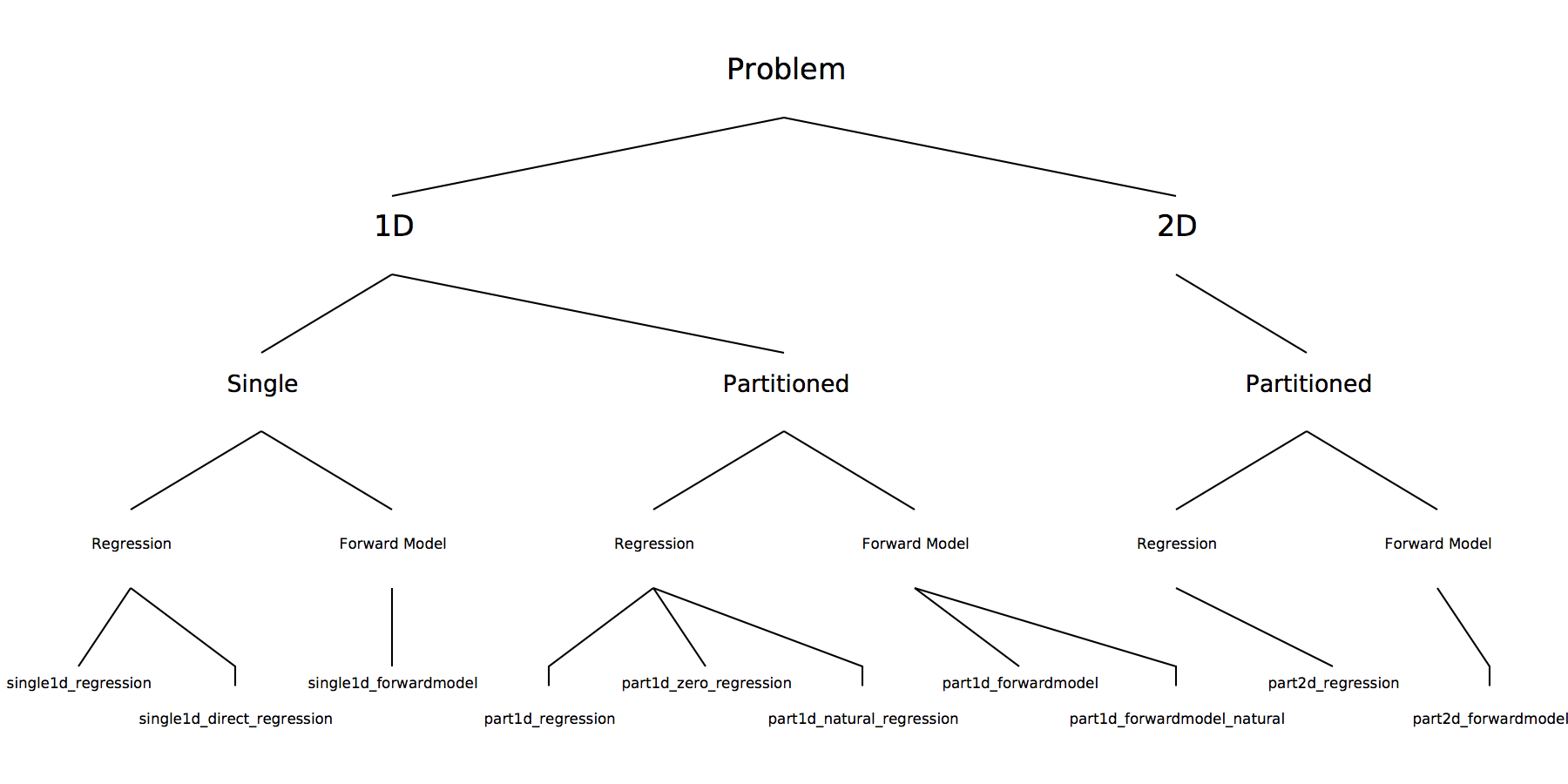## rj-MCMC

### Summary

This library provides routines for running Reversible Jump Monte-Carlo Markov chains for 1-D and 2-D spatial regression problems (i.e. given noisy x, y data, construct the underlying signal). It also allows generalization to any spatial 1-D and 2-D problem through the users inclusion of a forward model. The routines here are used as the basis of problem specific applications such as Receiver function inversion (code rj-RF) and 2-D travel time tomography (code rj-Tomo). For regression problems the method is also known as Bayesian Partition Modeling (Dension et al. 2002, Gallagher et al. 2011).

The regression problem is treated as one of Bayesian inference and sampled with transdimensional Markov chain. The parametrization of the unknown curve is variable and forms part of the inference process. The x-axis is divided into a set of partitions within each the data is fit with a polynomial with variable order (see Figure). With the package one can re-construct signals from noisy (x,y) data with the number of partitions, the location of the partition boundaries, the order of the polynomial in each partition, and the level of noise on the data (y values) all treated as unknown variables.The output of the algorithm is an ensemble of curves with varying numbers of partitions and (optionally) polynomial orders. By taking the mean, median or maximum of the PDF at each x point, an estimated ‘mean’ curve can be constructed from the ensemble of solutions. An example is given in the figure below.There are 8 different callable routines in the library, each callable from Fortran90, C or python. Each routine handles a specific problem setup. The Figure below describes the range of generic problems that can be dealt with.